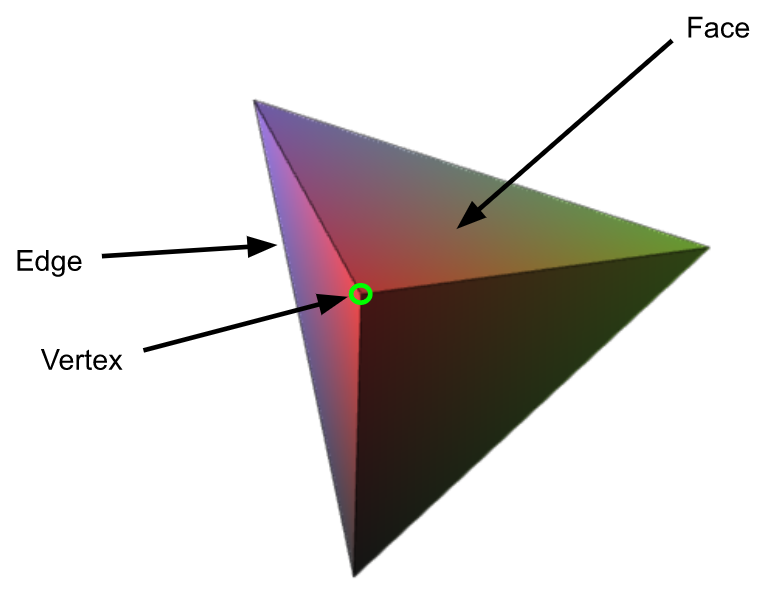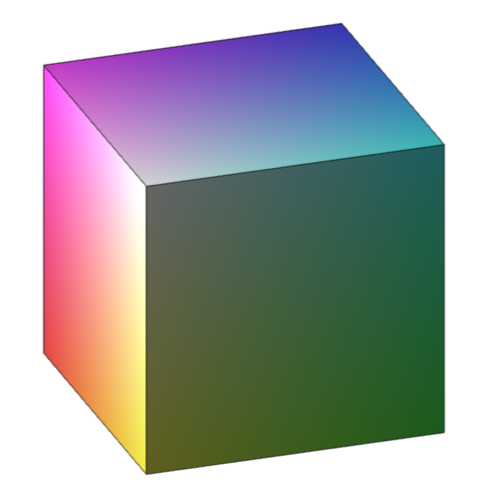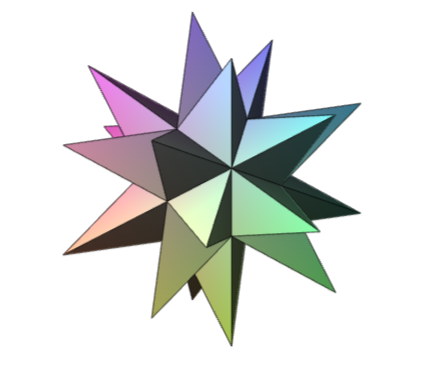## Regular Polyhedra and Schläfli Symbols

Maple Learn

When introduced to geometry, one of the first things we learn is the definition of the word “polygon”. A polygon is a closed 2-dimensional shape with at least 3 straight sides and angles. A regular polygon is a polygon with congruent sides and equal angles. A regular polygon with n sides has Schläfli symbol {n}. I’m interested in mathematical history, so when I learned that the idea of higher-dimensional spaces was invented in the middle of the nineteenth century I decided to research more about Ludwig Schläfli and the notation he came up with to describe his ideas.

In general, the Schläfli symbol is a notation of the form {p, q, r, ...} for regular polytopes. Polytopes are geometric objects with flat sides. This week, I will be focusing on 3-dimensional polytopes, also called polyhedra.

Similar to regular polygons, regular polyhedra are 3-dimensional shapes whose faces are all the same regular polygon. A regular polyhedron’s Schläfli symbol is of the form {p, q}, where p is the number of edges each face has and q is the number of faces that meet at each of the polyhedron’s vertices.Below are two regular polyhedra: a cube (also known as a hexahedron) and a great stellated dodecahedron. The cube is one of five Platonic solids, and the great stellated dodecahedron is one of four Kepler-Poinsot polyhedra – all of these can be represented by Schläfli symbols. The cube has Schläfli symbol {4, 3}, since squares have 4 equal sides, and each vertex of a cube is created by the vertices of 3 squares meeting.Can you figure out the Schläfli symbol for the great stellated dodecahedron?The great stellated dodecahedron has the Schläfli symbol {5/2, 3}. This is because great stellated dodecahedrons are regular star polygons. As a result, the first number in their Schläfli symbol is an irreducible fraction whose numerator represents a number of sides and whose denominator corresponds to a turning number. The particular fraction 5/2 corresponds to a pentagram – a regular star polygon with 5 points – and great stellated dodecahedrons are composed of 12 of these pentagrams, where 3 pentagrams meet at each vertex of the shape.

One notable example of a regular polytope in pop culture is the tesseract, which has the Schläfli symbol {4, 3, 3}. This is an extension of the cube’s Schläfli symbol, {4, 3}, and the last number indicates that there are three cubes folded together around every edge. Below are two representations of a tesseract: one that uses a Schlegel diagram (left) and one from the 2012 movie Avengers (right).

Try out our Regular Polyhedra Visualization Using Schlafli Symbol Notation! In this document, you can test out your own Schläfli symbols for regular polyhedra. If they are valid Schläfli symbols, you’ll be provided with a 3-D visualization of the shape. If they are invalid, you can check out the logic for finding the specifications for regular polyhedra and this document, which provides all the 3-D regular polyhedra for you to try out.﻿